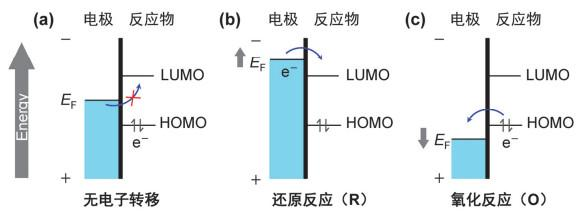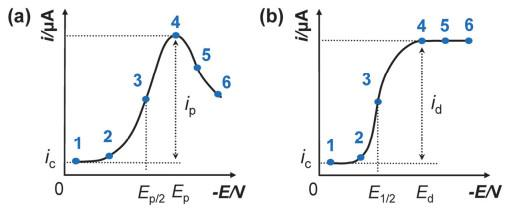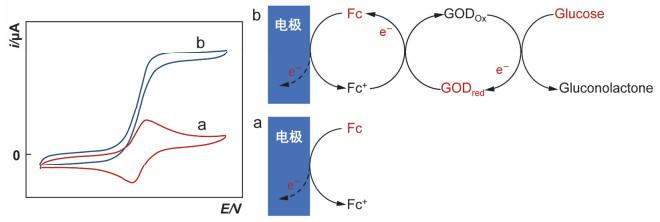## Principles of Linear Sweep Voltammetry and Interpretations of VoltammogramsAbstract

In this paper, we discussed the basic elements which affect the shape of linear sweep voltammograms in the reaction process on the electrode surface, that is, heterogeneous electron transfer and mass transfer in liquid phase. Derived from the basic definition and principle of voltammetry, the "supply-demand" mode between electron and mass transfer determines the characteristics of voltammograms. A series of voltammetric behaviors under different electrochemical testing conditions were discussed, including conventional electrodes and microelectrodes, reversible and irreversible electrode processes, stationary and rotating disk electrodes, and electrocatalysis. The basic laws that affect the shape of voltammograms provide a reference for understanding the fundamental principles of linear sweep voltammetry and data analysis.

Keywords： Principle of linear sweep voltammetry ; Electrode reaction process ; Interpretation of voltammograms ; Macro-and ultramicro-electrodes ; Reversibility ; Rotating disk electrode ; Electrocatalytic reaction

Hu Chengguo. Principles of Linear Sweep Voltammetry and Interpretations of Voltammograms. University Chemistry[J], 2021, 36(4): 2005071-0 doi:10.3866/PKU.DXHX202005071

### 图1### 图2#### 1.3 基本过程

(1) 电子在电极导体上的转移或输运(即电极导体上电子的输运)；

(2) 电子跨过两相界面的异相电子转移过程(即两相界面物质的消耗)；

(3) 物质从溶液本体向电极|溶液界面的液相传质过程(即溶液中物质的供应)。

### 图3### 图4#### 1.3.2 液相传质过程——物质的供应

$\text { 暂态 } \frac{\partial J_{x, t}}{\partial t}=\frac{\partial c(x, t)}{\partial x}=D \frac{\partial^{2} c(x, t)}{\partial x^{2}}$

$\text { 稳态 } \quad J_{x, t}=-D \frac{\partial c}{\partial x}$

### 图5### 图6### 图7### 图8### 图9$\text { Glucose }+\mathrm{GOD}_{\text {ox }} \rightarrow \text { Gluconolactone }+\mathrm{GOD}_{\text {red }}$

$\mathrm{GOD}_{\mathrm{red}}+2 \mathrm{Fc}^{+} \rightarrow \mathrm{GOD}_{\mathrm{ox}}+2 \mathrm{Fc}+2 \mathrm{H}^{+}$

$2 \mathrm{Fc} \rightleftharpoons 2 \mathrm{Fc}^{+}+2 \mathrm{e}^{-}$

## 参考文献 原文顺序 文献年度倒序 文中引用次数倒序 被引期刊影响因子

Masek A. ; Chrzescijanska E. ; Zaborski M. Electrochim. Acta 2013, 107, 441.

McGaw A. E. ; Swain G. M. Anal. Chim. Acta 2006, 575, 180.

Bard, A. J.; Faulkner, L. R. 电化学方法原理和应用. 第2版. 邵元华, 朱果逸, 董献堆, 张柏林, 译. 北京: 高等教育出版社, 2005: 3.

Reiss H. J. Phys. Chem. 1985, 89, 3783.

Heinze J. Angew. Chem. Int. Ed. Engl. 1984, 23, 831.

Dayton M. A. ; Brown J. C. ; Stutts K. J. ; Wightman R. M. Anal. Chem. 1980, 52, 946.

Heinze J. Angew. Chem. Int. Ed. Engl. 1991, 30, 170.

Paulus U. A. ; Schmidt T. J. ; Gasteiger H. A. ; Behm R. J. J. Electroanal. Chem. 2001, 495, 134.

Qiu J. D. ; Zhou W. M. ; Guo J. ; Wang R. ; Liang R. P. Anal. Biochem. 2009, 385, 264.

Cass A. E. G. ; Davis G. ; Francis G. D. ; Hill H. A. O. Anal. Chem. 1984, 56, 667.

/

 〈〉